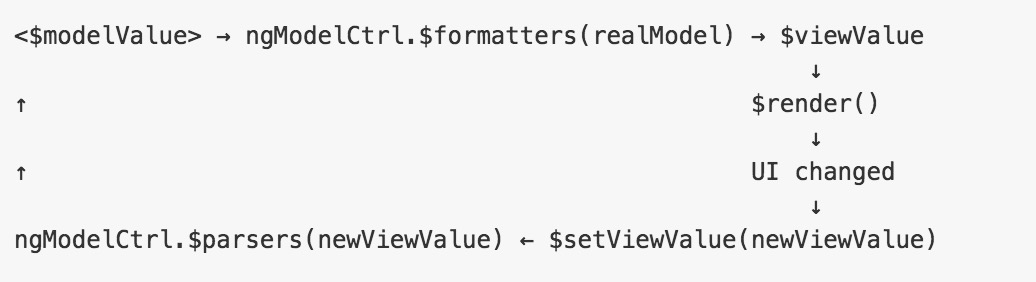Angular Input格式化

0
0
01. 云栖社区>
2. 博客>
3. 正文

Angular Input格式化

ghost丶桃子 2016-05-23 17:33:45 浏览725

<input type="text" ng-model="demo.text | uppercase" />.directive('textTransform', [function() {

return {
require: 'ngModel',
link: function(scope, element, iAttrs, ngModelCtrl) {
ngModelCtrl.\$parsers.push(function(value) {
...
});

ngModelCtrl.\$formatters.push(function(value) {
...
});
}
};
}]);

.directive('textTransform', function() {
var transformConfig = {
uppercase: function(input){
return input.toUpperCase();
},
capitalize: function(input){
return input.replace(
/([a-zA-Z])([a-zA-Z]*)/gi,
function(matched, \$1, \$2){
return \$1.toUpperCase() + \$2;
});
},
lowercase: function(input){
return input.toLowerCase();
}
};
return {
require: 'ngModel',
link: function(scope, element, iAttrs, modelCtrl) {
var transform = transformConfig[iAttrs.textTransform];
if(transform){
modelCtrl.\$parsers.push(function(input) {
return transform(input || "");
});

element.css("text-transform", iAttrs.textTransform);
}
}
};
});

<input type="text" ng-model="demo.text" text-transform="capitalize" />
<input type="text" ng-model="demo.text" text-transform="uppercase" />
<input type="text" ng-model="demo.text" text-transform="lowercase" />

ghost丶桃子
+ 关注

corcosa 16170人浏览